Trigonometry Basics
 Home > Lessons > Trig Basics Search | Updated October 20th, 2016
Introduction

In this section, you will learn the basics of triangles in order to do trigonometry problems. Here are the sections within this lesson:

 Labeling Triangles We will be looking at three-sided polygons, called triangles. Triangles have three sides and three angles.The angles are located at the corners of the triangle, which are called vertices. The sides are the segments between the angles.The next step involves labeling the vertices (angles) of the triangle. Traditionally, we label the right angle with the letter 'C.' However, with this generic triangle, we will label the angles 'A,' 'B' and 'C.' Notice that we use capital letters when labeling angles.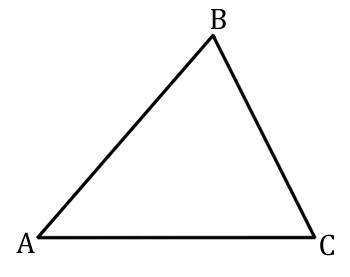When we label the sides, we place small case letters opposite their corresponding angles. For instance, the letter 'a' will be opposite angle 'A.'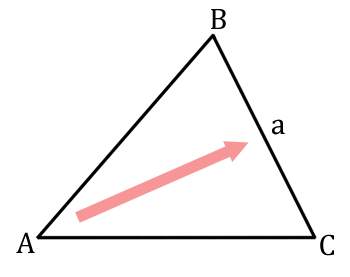Side 'b' will be opposite angle 'B.'Side 'c' will be opposite angle 'C.'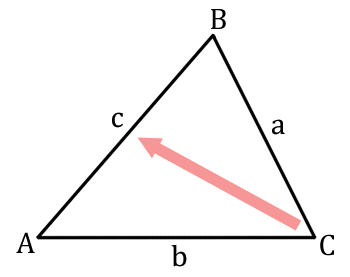This leaves us with our completely labeled triangle.The Three Main Trigonometric Functions There are three main trigonometric functions: sine, cosine, and tangent. The definitions of those functions can be found in the next graphic. The Greek letter theta has been used to describe and them is typically used when describing the focus angle.These functions are used a considerable number of times in order to solve lengths of sides and measures of angles within right triangles. To help remember the definitions for the functions, many people abbreviate the definitions. Let's look at using 'O' for 'opposite, 'A' for adjacent, and "H" for hypotenuse. The definitions would then look like this.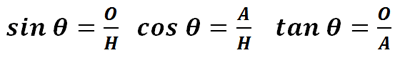To further abbreviate, look at the following highlighted portions of the definitions.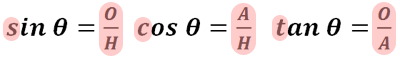The 'S' with sine links together with 'O' and 'H' to form 'SOH.' Likewise, the letters 'CAH' go together as do 'TOA.' This leads us to a commonly used mnemonic device.This mnemonic device allows us to easily remember the definitions of these trigonometric functions. So that we can see how they are used on triangles, let's look at a right triangle.We will focus on angle 'A' for the purposes of labeling the triangle's sides 'opposite,' 'adjacent,' and 'hypotenuse.' The opposite side of the triangle is the side furthest from the angle we are examining. Look at this graphic to find the opposite side of the triangle.The hypotenuse of a triangle is always the side furthest from the right angle. So, the hypotenuse sits opposite angle 'C' in this diagram.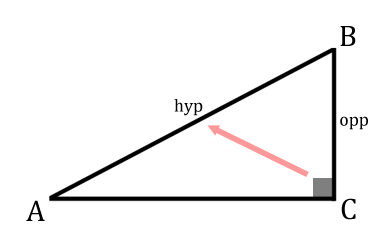Last, we have the remaining side, called the adjacent side of the right triangle. This is the side next to the angle in focus, as seen here.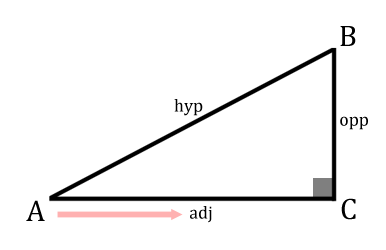This leaves us with the following triangle as it is viewed from angle 'A.'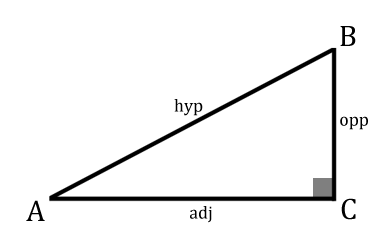Three Other Trigonometric Functions Besides the three main trigonometric functions, there are three other trigonometric functions: cosecant, secant, and cotangent. The definitions of those functions can be found in the next graphic. The Greek letter theta has been used to describe and them is typically used when describing the focus angle.To remember these three functions, let's see how they relate to the first group of three functions. When we look at the definitions, we can see that sine and cosecant are closely related. They are reciprocal functions. Likewise, cosine and secant are reciprocal functions and tangent and cotangent are reciprocal functions, too.    Tangent and cotangent sound the same; so, they are easily connected to each other.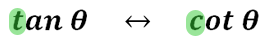As for the other four, we can see that reciprocal functions can be remembered by looking at their first letters.It can be seen that the 's' from sine connects mathematically with 'c' from 'cosecant.' Similarly, the 'c' from 'cosine' goes together with the 's' from 'secant.'    Another way to remember the new functions is to use a mnemonic device, like this one. Look at the definitions of the new trigonometric funtions.Highlighting the first letters of the functions and their ratios will be used to form a new mnemonic device.Like SOH CAH TOA is used for the three main trigonometric functions, this is the mnemonic device for the other three trigonometric functions.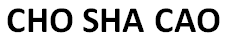Deriving 30-60-90 Relationships This video will derive the relationship between the sides and angles of a 30-60-90 triangle.ideo: Deriving The 30-60-90 Special Right Triangle Deriving 45-45-90 Relationships This video will derive the relationship between the sides and angles of a 45-45-90 triangle.ideo: Deriving The 45-45-90 Special Right Triangle Related Lessons We would like to invite you to learn from our advanced trigonometry sections. In these sections, you can learn details that require their own lesson pages.esson: Right Triangle Trigonometryesson: Trigonometric Anglesesson: The Law of Sinesesson: The Law of Cosinesesson: Trigonometric Expressions